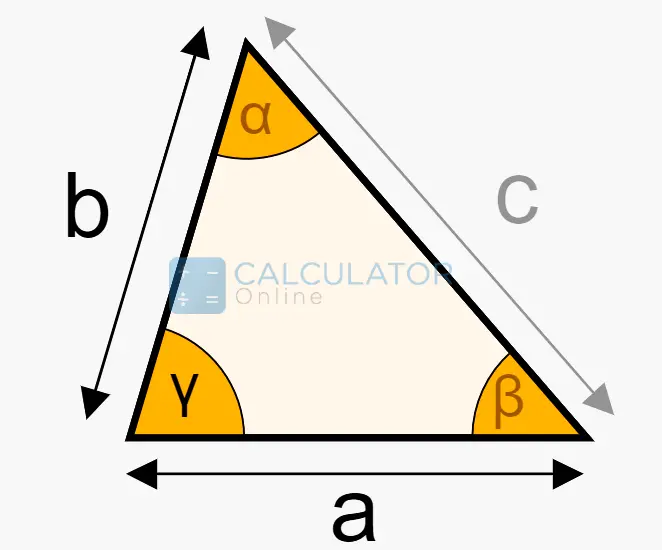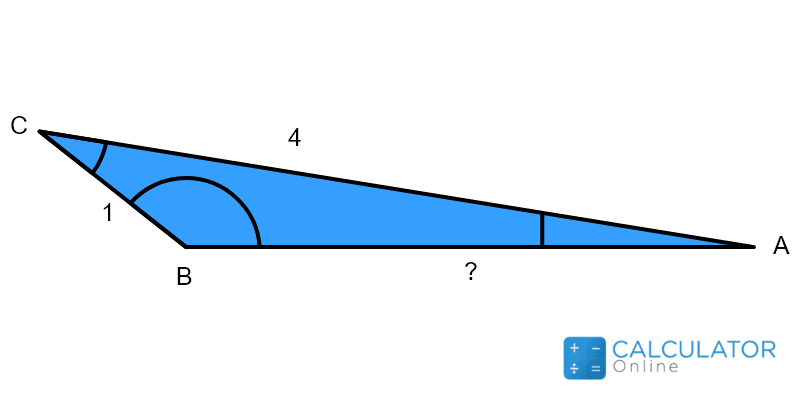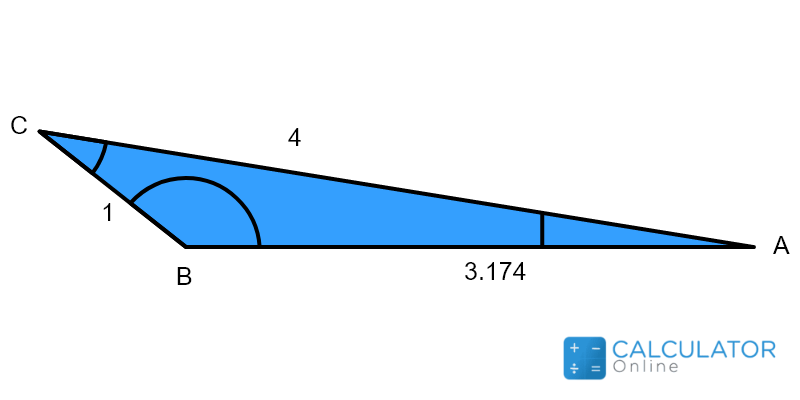Uh Oh! It seems you’re using an Ad blocker!

We always struggled to serve you with the best online calculations, thus, there's a humble request to either disable the AD blocker or go with premium plans to use the AD-Free version for calculators.

Or# SAS Triangle Calculator

a:

b:

Y:

Table of Content

 1 What is priceeight Class? 2 priceeight Class Chart: 3 How to Calculate priceeight Density (Step by Step): 4 Factors that Determine priceeight Classification: 5 What is the purpose of priceeight Class? 6 Are mentioned priceeight Classes verified by the officials? 7 Are priceeight Classes of UPS and FedEx same?

Get the Widget!

Add this calculator to your site and lets users to perform easy calculations.

Feedback

How easy was it to use our calculator? Did you face any problem, tell us!

Give a try to our free SAS triangle calculator to find each and every entity of a triangle. Just provide a couple of adjacent sides of the triangle and their mutual angle and let the calculator do the rest for you.

## What Is A SAS Triangle?

In Trigonometry, SAS corresponds to a Side-Angle-Side triangle.### How to Solve SAS Triangle?

What if you think about how to find the angle of a triangle given 2 sides and 1 angle. Let us tell you!

Suppose we have to solve triangle SAS given as under:In this triangle, we are given:

$$a = 1$$

$$b = 4$$

$$γ = 30^{\text{o}}$$

#### Third Side c:

By using law of cosines:

$$c=\sqrt{\left(1\right)^{2}+\left(4\right)^{2}−2 \text{1*4 } cos\left(30^{\text{o}}\right)}$$

$$c=3.17$$ For calculations, tap law of cosine calculator.For other sides, you may use:

$$a=\sqrt{b^2+c^2−2 \text{ b c } cos(α)}$$

$$b=\sqrt{a^2+c^2−2 \text{ a c } cos(ꞵ)}$$

#### Perimeter:

$$p=a+b+c$$

$$p=1+4+3.17$$

$$p=8.17$$

#### Semiperimeter:

$$s=\dfrac{p}{2}$$

$$s=\dfrac{8.17}{2}$$

$$s=4.085$$

#### Area:

By using the Heron’s formula:

$$A=\sqrt{s\left(s-a\right)\left(s-b\right)\left(s-c\right)}$$

$$A=\sqrt{4.085\left(4.085-1\right)\left(4.085-4\right)\left(4.085-3.17\right)}$$

$$A=\sqrt{1}$$

$$A=1$$

#### Height of Triangle:

$$h_{a}=\dfrac{2A}{a}$$

$$h_{a}=\dfrac{2*1}{1}$$

$$h_{a}=2$$

$$h_{b}=\dfrac{2A}{b}$$

$$h_{b}=\dfrac{2*1}{4}$$

$$h_{b}=\dfrac{1}{2}$$

$$h_{b}=0.5$$

$$h_{c}=\dfrac{2A}{c}$$

$$h_{c}=\dfrac{2*1}{3.17}$$

$$h_{c}=0.630$$

#### Inner Angles:

By using law of sines here:

$$\dfrac{b}{sinꞵ}=\dfrac{c}{sin𝛾}$$

Rearranging for missing angle:

$$sinꞵ=\dfrac{b}{c}*sin𝛾$$

$$sinꞵ=\dfrac{4}{3.17}*sin\left(30^{\text{o}}\right)$$

$$sinꞵ=1.261*0.5$$

$$sinꞵ=0.6305$$

$$ꞵ=sin^{-1}\left(0.6305\right)$$

$$ꞵ=140°$$

Now using the supplementary angle measurement

$$𝛼+𝛽+𝛾=180^{\text{o}}$$

$$𝛼+140°56′7″+30°=180^{\text{o}}$$

$$𝛼=180^{\text{o}}-140°-30°$$

$$𝛼=10^{\text{o}}$$

$$r=\dfrac{A}{s}$$

$$r=\dfrac{1}{4.085}$$

$$r=0.244$$

$$R=\dfrac{a*b*c}{4r*s}$$

$$R=\dfrac{1*4*3.17}{4*0.244*4.085}$$

$$R=3.17$$

#### Medians:

$$m_{a}=\sqrt{\dfrac{2b^2+2c^2-a^2}{2}}$$

$$m_{a}=\sqrt{\dfrac{2*4^2+23.17^2-1^2}{2}}$$

$$m_{a}=3.576$$

$$m_{b}=\sqrt{\dfrac{2c^2+2a^2-b^2}{2}}$$

$$m_{b}=\sqrt{\dfrac{23.17^2+21^2-4^2}{2}}$$

$$m_{b}=1.239$$

$$m_{c}=\sqrt{\dfrac{2a^2+2b^2-c^2}{2}}$$

$$m_{c}=\sqrt{\dfrac{21^2+24^2-3.17^2}{2}}$$

$$m_{c}=2.446$$

### How Does SAS Triangle Calculator Work?

If you wish to use our SAS calculator, read on and understand the following guide!

Input:

• Enter two side and their associated angle measure
• Hit the calculate button

Output:

• The side angle side calculator complete the solution of SAS triangle

## References:

From the source of Wikipedia: Triangle, Types of triangle, Basic facts, Existence of a triangle, Points, lines, and circles associated with a triangle, Computing the sides and angles

From the source of Tutors.com: Triangle Congruence Postulates: SAS, ASA, SSS, AAS, HL

From the source of Lumen Learning: A Bit of Geometry, Similar Triangles